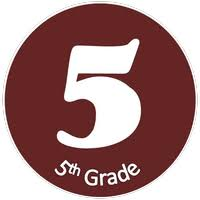Price \$35/Session
Duration 1 Session
Credit Week

Course Description

Course Objective:

The students will be instructed and given practice in basic mathematical operations and problem-solving strategies in the areas of addition, subtraction, multiplication, and division of whole numbers, decimals, fractions, ration and proportion, and geometry.

Textbook:

• Math 5, Pearson enVision, Copyright 2017

Materials:

•  Student Textbook & homework book
•  Problem of the Day
•  CD / DVD support
•  Practice and Enrichment Worksheets
•  Manipulative materials
•  Calculators
•  Assessment Sourcebook

Time Allotment:

• 45 minutes per day, 2 days per week

Course Content:

• Data, Graphs, and Facts Review
• Whole Numbers and Decimals: Place Value, Adding, and Subtracting
• Multiplying Whole Numbers and Decimals
• Dividing Whole Numbers and Decimals – 1-Digit Divisors, 2-Digit Divisors
• Geometry – angles, polygons, length, perimeter, area
• Fractions – mixed numbers, adding, subtracting, multiplying, dividing

Measurement:

• Ratio, Percent, Probability

Areas to be evaluated:

•  Class participation
•  Homework assignments
•  Tests
•  Quizzes